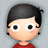#### 学习笔记TF002: 怎么安装TensorFlow

tinyfool 等1人欣赏。

TensorFlow需要用到两个经典库：Jupyter(iPython) Notebook、matplotlib。Jupyter Notebook可以交互式编写可视化结果文档，代码展示，Markdown单元，设计原型，代码写入逻辑块，方便调试脚本特定部分。matplotlib是绘图库，可以实现数据可视化，典型应用Seaborn。

Virtualenv环境安装（看网络情况，我装了四小时，重试了无数次这两个命令，尤其是第二个） sudo easy_install pip sudo pip install --upgrade virtualenv

import tensorflow as tf import numpy as np import matplotlib.pyplot as plt

%matplotlib inline a = tf.random_normal([2,20]) sess = tf.Session() out = sess.run(a) x,y = out

plt.scatter(x,y) plt.show()